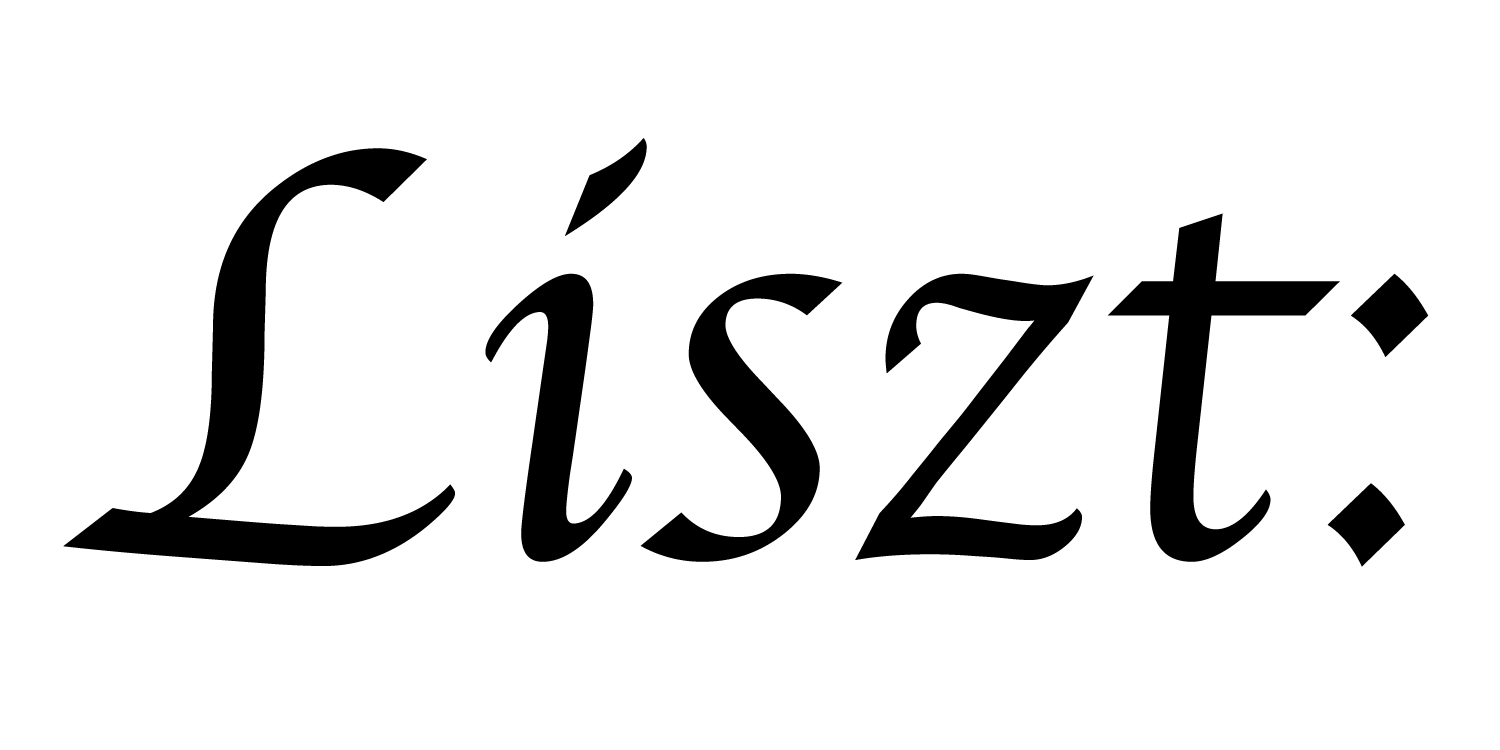Except in the case of grids, we’ll want to load the domain data from a file. This example program demonstrates loading an octahedron triangle mesh.

``````import 'ebb'
local L = require 'ebblib'

local ioOff = require 'ebb.domains.ioOff'
``````

This time we load the `ioOff` domain library instead of the `grid` domain library. OFF is a simple file format for triangle meshes, and `ioOff` defines a wrapper around the standard triangle mesh library to load data from OFF files. Once we have the library required, we use the library function `LoadTrimesh()` to load an octahedron file. Unfortunately, because of how we specify the filepath here, this example can only be executed successfully from `LISZT_EBB_ROOT`. Instead, let’s do something more robust and only slightly more complicated.

``````local ioOff = require 'ebb.domains.ioOff'
local PN    = require 'ebb.lib.pathname'
local mesh  = ioOff.LoadTrimesh( PN.scriptdir() .. 'octa.off' )
``````

In this version we load the Ebb-provided `pathname` library to help us manipulate filesystem paths. Using this library, we can introspect on where this particular program file is located on disk (`PN.scriptdir()`) and reference the OFF file from there. Now this script can be run safely from anywhere.

``````print(mesh.vertices:Size())
print(mesh.edges:Size())
print(mesh.triangles:Size())
``````

Having loaded the octahedron, let’s print out some simple statistics about how many elements of each kind it contains. If everything is working fine, we should expect to see `6`, `24`, and `8`. (Why 24? The standard triangle mesh represents directed rather than undirected edges.)

``````mesh.vertices.pos:Print()
``````

When we load in the triangle mesh, the vertices are assigned positions from the file. Here, we print those out to inspect. They should be unit distance away from the origin along each axis. Take a look at the OFF file itself (it’s in plaintext) and you’ll see that the positions there correspond to the positions printed out.

``````local ebb translate ( v : mesh.vertices )
v.pos += {1,0,0}
end

mesh.vertices:foreach(translate)

mesh.vertices.pos:Print()
``````

Finally, we can write an Ebb function to translate all of the vertices, execute it, and then print the results.

a part of the Liszt project and PSAAP II center at Stanford University